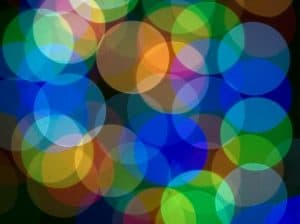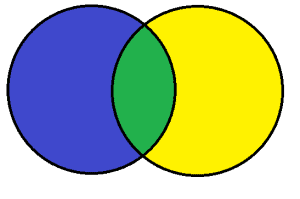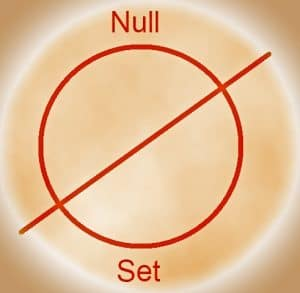# Sets & Set Theory

Students are usually introduced to the concept of sets and set theory at a relatively young age. This introduction, however, may not happen in a math class or be introduced as a separate branch of mathematics. In fact, most people probably first encountered set theory through Venn diagrams of some sort.

These diagrams may have popped up in a science class for animal classification. For example, one circle may contain “animals that fly” and the other side may include “mammals.” The overlap in the middle would include bats. Alternatively, a mathematical version of a Venn diagram may have had even numbers in one circle and prime numbers in another. Their overlap would include the number 2.

Students may also be familiar with some mathematical problems that involve Venn diagrams or that can be represented with one.

For instance, suppose there are 20 students who play either hockey, basketball, or both. If 10 play hockey and 5 play basketball, how many play both?Set theory builds off of these familiar models to create a system that is useful in mathematics, philosophy, and logic.

For example, subsets can be used to illustrate necessary and sufficient causes. Many mathematical researchers work with sets on a daily basis and try to prove theories relating to them.

This guide introduces sets and how to describe them. That topic gives the basics needed to express ideas in set notation. There are also resources on Venn diagrams, the null set, subsets, and complements.

This guide also includes a second section with information on set operations. That topic discusses how to combine sets beginning with examples of two sets and three sets. It ends by discussing how to illustrate the concepts.

## Introduction to Sets

While the concept of “set” may seem unfamiliar, most people use sets every day without calling it set theory. For instance, many people have a mental list of stores that they use to decide where to shop. If someone needs just eggs, they may go to a convenience store. If they need just shampoo, they may go to a pharmacy. If the person needs both eggs and shampoo, however, they would probably have to go to a large supermarket if they wanted to make just one stop.

Alternatively, most people probably have a mental list of places they go to meet with friends and places they go to study or get work done. Perhaps the park is only for friends and the office is only for work while a café works for both.These are the basic ideas behind set theory. This section aims to explore these concepts as they relate to mathematical structures.

This topic begins by explaining how to describe sets. It goes on to note the difference between finite and infinite sets. Next, it introduces the null set, which is the set that contains nothing. The resources also explain Venn diagrams and subsets, which are smaller parts of a larger set. The topic ends by explaining the universal set and set complements.

• Describing Sets
• Set Notation
• Finite and Infinite Sets
• Empty Set or Null Set
• Set Equality
• Venn Diagrams
• Subsets
• Universal Set
• Complement of a Set

## Set Operations

Sets can be compared through operations, just like numbers can be compared through operations. Of course, when discussing sets, the operations do not follow the lines of traditional addition, subtraction, multiplication, and division.Instead, set operations focus on intersections, which are the overlaps of sets, and unions, which are the combination of two sets. Intersections are indicated verbally by the word “and.” For instance, numbers that are even and composite would be the intersection of the sets “even numbers” and “composite numbers.” This intersection would be an infinite set that contained all even numbers except 2.

Unions are represented by the word “or”. It is important to note that in math, “or” is not exclusive like it is in everyday English. The mathematical “or” includes “both”. For instance, the set of numbers that are prime or odd would include the numbers 2, 3, and 9.

This topic also talks about the complement of sets, which is essentially the universal set, which contains everything, minus a given set.

This topic begins by explaining intersections of two sets and intersections three sets. It also introduces complements and unions before describing how to combine set operations. The topic ends with subtopics explaining how to represent these set operations.

• Intersection of Two Sets
• Intersection of Three Sets
• Complement of an Intersection
• Union of Sets
• Combined Operations of Sets
• Drawing Venn Diagrams
• De Morgan’s Theorem